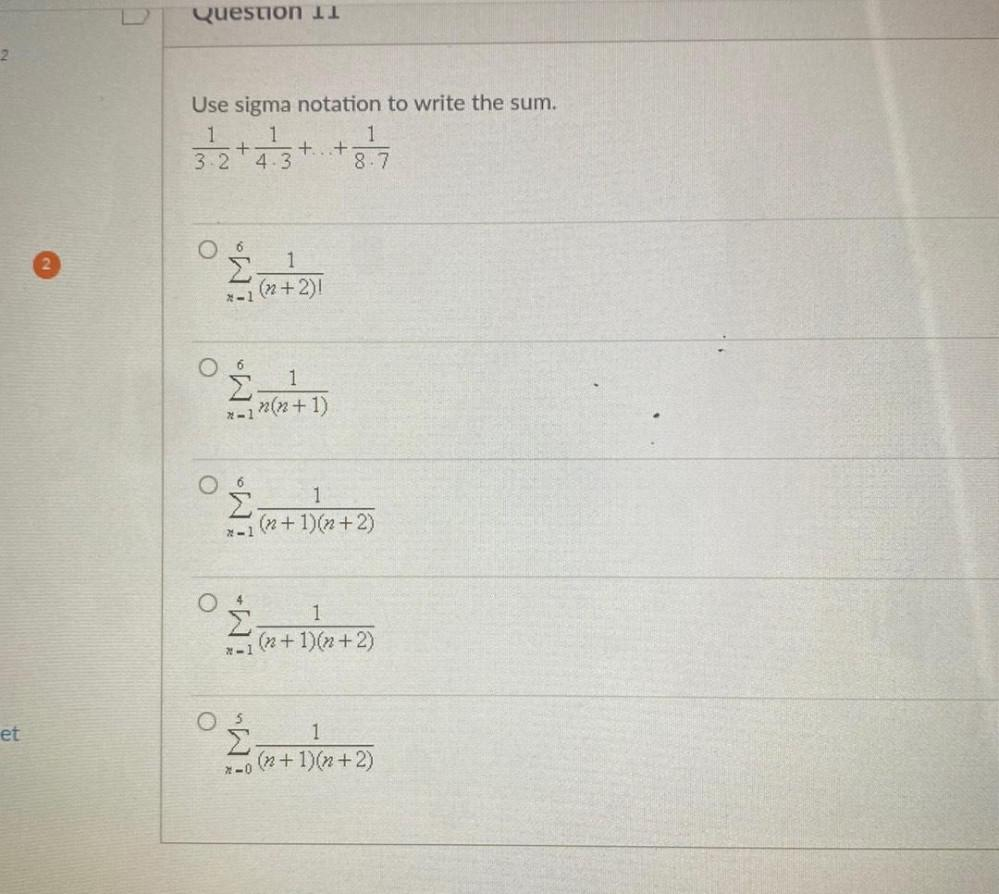Question:

# Question 11 2 stattuta Use sigma notation to write the sum. 1 1 1 + +...+ 3.2 4.3 8.7 6 º puta 1 x-1 (n+2)! 6 ° months x-1 n(n+1Question 11 2 stattuta Use sigma notation to write the sum. 1 1 1 + +...+ 3.2 4.3 8.7 6 º puta 1 x-1 (n+2)! 6 ° months x-1 n(n+1) ° 3 + 14+ 1 (n + 1)(x+2) 4 ° m+ )+) 1 x-1(n + 1)(n+2) et ° 1)x+2) x-0 (n+1)(n+2)